Science, Maths & Technology

### Become an OU student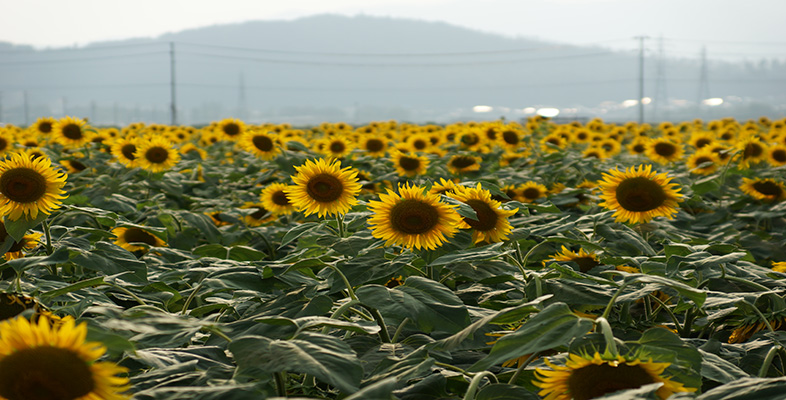Ratio, proportion and percentages

Start this free course now. Just create an account and sign in. Enrol and complete the course for a free statement of participation or digital badge if available.

# 1.4.1 Try some yourself

## Activity 5

Convert each of the following fraction ratios to decimal ratios.

• (a)• (b)• (c)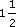• (d)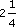• (a)= 0.2

• (b)= 0.3333 ... this repeats indefinitely. As a decimal, you need to terminate after some finite number of places, e.g. 0.3333 to four decimal places.

• (c)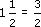= 1.5

• (d)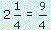= 2.25

Alternatively, 2 is 2.00,is 0.25, and adding them together gives 2.25.

## Activity 6

Convert each of the following decimal ratios to fraction ratios.

• (a) 0.25

• (b) 1.135

• (c) 0.064

• (a) 0.25 =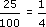• (b)1.135 =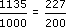• (c)0.064 =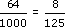## Activity 7

A recipe for a casserole involves soaking dried beans. The beans require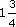litres of water per kilogram of beans. If you have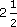kilograms of beans, how much water is required?

You need to multiplyby. First convert to top-heavy fractions or decimals: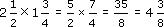So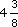or 4.375 litres of water is required.